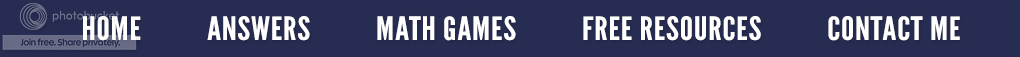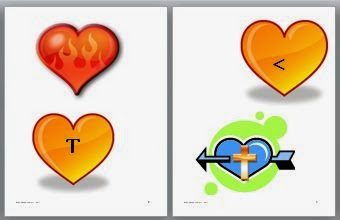### Magically Squaring Numbers

My college math students lack confidence (I classify them as mathphobics.); so, I like to show them math "tricks" which they can use to impress their peers.  I encourage them to know their squares through 25. (Yes, I know they can use a calculator, but the mind is so much quicker!)  When we get to solving equations using the Pythagorean Theorem, I introduce this trick. Please note: For the trick to work, it must be a two digit number that ends in 5.
Suppose we have 352.  (This means will be making a square.)
This means that we can build a square that is 35 by 35, and it will contain 1,225 squares or have an area of 1225 squares.

Now let's try 652
• One more than 6 is 7; so, 6 x 7 is 42.
• Place 42 in front of 25 (5 x 5) and so 65 squared is 4,225.
• 6 × 7 = 42      5 × 5 = 25
How about finding the square root? We begin by looking at the numbers in the thousands and hundreds place. In the answer of 1,225, we would use the 12. Think of the factors of 12 that are consecutive numbers. In this case, they would be 3 and 4. Use the smaller of the two which, in this case, is 3. Now place a five after it. You now know the square root of 1,225 is 35.
Thirty-five represents the length of one of the sides of a square that contains 1,225 squares.

Now, try some numbers on your own. When you get comfortable with the "trick", try it with your students. They will find out that math can be magical!

### A Perfect Ten - Why Our Number System is Called Base Ten

Don't you love tests where you ask a question which you believe everyone will get correct, and then find out it just isn't so?  I gave my algebra college students a pretest to see what they knew and didn't know.  One of the first questions was:  Why is our number system called Base Ten?  This is an extremely important concept as it reveals what they know about place value.  Below are some of the answers I received.

1)  It is called Base Ten because we have ten fingers.  (Yikes! If that is so, should we include our toes as well?)

2)  It is called Base Ten because I think you multiply by ten when you move past the decimal sign.  (Well, sort of.  You do multiply by ten when you move to the left of the decimal sign, going from the ones place, to the tens place, to the hundreds place, etc.)

3)  I think it is called Base Ten because it's something we use everyday.  (Really????)

Enough!  It is called Base Ten because we use ten digits (0, 1, 2, 3, 4, 5, 6, 7, 8, and 9) to write all of the other numbers.  Each digit can have one of ten values: any number from 0 through 9. When the value reaches 9, just before 10, it starts over at zero again.  (Notice the pattern below.)

0, 1, 2, 3, 4, 5, 6, 7, 8, 9, 10, 11, 12, 13, 14, 15, 16, 17, 18, 19, 20, etc.

In addition, each place is worth ten times more than the last. Ten is worth ten times more than 1, and 1,000 is ten times more than 100. The pattern continues infinitely both ways on a number line.

The decimal point allows for the place value to continue in a consistent pattern with numbers smaller than one. As we move to the right of the decimal point, each place is divided by ten to get to the next place value. One hundredth is one tenth divided by ten, and one thousandth is one hundredth divided by ten. The pattern goes on infinitely.

100's, 10's, 1's . 0.1, 0.01, 0.001, 0.0001, 0.00001, etc.Since all mathematics is based on patterns, this should not be a new revelation. Perhaps on the post-test, my students will omit the fingers and instead rely on patterns to answer the questions!

### Learn by Heart - Heart Rebus Puzzles

Valentine's Day is fast approaching. Here is a fun February activity which uses heart rebus puzzles. It is called Hearts and Valentines. This resource is 13 pages and features 24 heart rebuses (idioms) that represent familiar expressions that contain the word "heart". (e.g. From the Bottom of My Heart or Cross My Heart) Each illustration uses a picture or symbol to represent a word or phrase. The students must use logic and reasoning skills to solve the 24 rebuses (idioms).

Each day during the month of February, put up one heart illustration as a student focus activity, OR, if you choose, place two or three up at one time or display all of them up at the same time. Students are to figure out which heart expression each heart picture represents. Below are four examples from the resource. Can you figure out the answers?Hearts and Valentines

I have stumped you? Perhaps you just don't have the heart to do it or maybe your heart simply isn't in it. If you give up, you'll find the answers on the page entitled Answers to Problems. (Look at the bar at the top of this blog post.)

As you have discovered, this can be a fun, but also very challenging Valentine's Day activity! If you would like more information about this resource, just click the title under the pictures above. And.... Happy Valentine's Day from the bottom of my heart!